# DEVELOPING THEMATIC HIERARCHIES FOR TEXT DOCUMENTS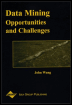Chapter VIII - Mining Text Documents for Thematic Hierarchies Using Self-Organizing Maps Data Mining: Opportunities and Challenges by John Wang (ed) Idea Group Publishing 2003Brought to you by Team-Fly

## DEVELOPING THEMATIC HIERARCHIES FOR TEXT DOCUMENTS

After the clustering process, each neuron in the DCM and the WCM actually represents a document cluster and a word cluster, respectively. Such clusters can be considered as categories in text categorization of the underlying corpus. In this section, we will describe two aspects of finding implicit structures of these categories. First, we will present a method for revealing the hierarchical structure among categories. Second, a category theme identification method is developed to find the labels of each category for easy human interpretation. The implicit structures of a text corpus can then be discovered and represented in a humanly comprehensible way through our methods.

### Automatic Category Hierarchy Generation

To obtain a category hierarchy, we first cluster documents by the SOM using the method described in last section to generate the DCM and the WCM. As we mentioned before, a neuron in the document cluster map represents a cluster of documents. A cluster here also represents a category in text categorization terminology. Documents labeled to the same neuron, or neighboring neurons, usually contain words that often co-occur in these documents. By virtue of the SOM algorithm, the synaptic weight vectors of neighboring neurons have the least difference compared to those of distant neurons. That is, similar document clusters will correspond to neighboring neurons in the DCM. Thus, we may generate a cluster of similar clusters or a super-cluster by assembling neighboring neurons. This will essentially create a two-level hierarchy. In this hierarchy, the parent node is the constructed super-cluster and the child nodes are the clusters that compose the super-cluster. The hierarchy generation process can be further applied to each child node to establish the next level of this hierarchy. The overall hierarchy of the categories can then be established iteratively using such top-down approach until a stop criterion is satisfied.

To form a super-cluster, we first define distance between two clusters: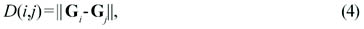where i and j are the neuron indices of the two clusters, and Gi is the two-dimensional grid location of neuron i. For a square formation of neurons, Gi = (i mod J1/2,i div J1/2). D(i,j) measures the Euclidean distance between the two coordinates Gi and Gj. We also define the dissimilarity between two clusters: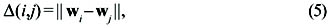where wi is the weight vector of neuron i. We may compute the supporting cluster similarity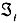for a neuron i from its neighboring neurons by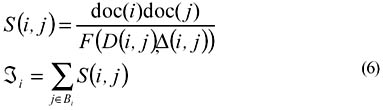where doc(i) is the number of documents associated to neuron i in the document cluster map, and Bi is the set of neuron index in the neighborhood of neuron i. The function F: R+R+ is a monotonically increasing function that takes D(i,j) and Δ(i,j) as arguments. The super-clusters are developed from a set of dominating neurons in the map. A dominating neuron is a neuron that has locally maximal supporting cluster similarity. We may select all dominating neurons in the map by the following algorithm:

1. Find the neuron with the largest supporting cluster similarity. Select this neuron as dominating neuron.

2. Eliminate its neighbor neurons so that they will not be considered as dominating neurons.

3. If there is no neuron left or the number of dominating neurons exceeds a predeter-mined value, stop. Otherwise go to Step 1.

The algorithm finds dominating neurons from all neurons under consideration and creates a level of the hierarchy. The overall process is depicted in Figure 2. As shown in that figure, we may develop a two-level hierarchy by using a super-cluster (or dominating neuron) as the parent node and the clusters (or neurons) that are similar to the super-cluster as the child nodes. In Figure 2, super-cluster k corresponds to the dominating neuron k. The clusters associated with those neurons in the neighborhood of neuron k are used as the child nodes of the hierarchy.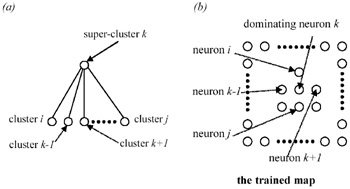Figure 2: (a) A two-level hierarchy comprises a super-cluster as root node and several clusters as child nodes. (b) The dominating neuron k is selected and used as a super-cluster. Its neighboring neurons compose the super-cluster. We only show a possible construction of the hierarchy here.

A dominating neuron is the centroid of a super-cluster, which contains several child clusters. We will use the neuron index of a neuron as the index of the cluster associated with it. For consistency, the neuron index of a dominating neuron is used as the index of its corresponding super-cluster. The child clusters of a super-cluster can be found by the following rule: The ith cluster (neuron) belongs to the kth super-cluster if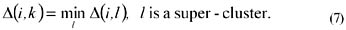The above process creates a two-level hierarchy of categories. In the following, we will show how to obtain the overall hierarchy. A super-cluster may be thought of as a category that contains several sub-categories. In the first application of the super-cluster generation process (denoted by STAGE-1), we obtain a set of super-clusters. Each super-cluster is used as the root node of a hierarchy. Thus the number of generated hierarchies is the same as the number of super-clusters obtained in STAGE-1. Note that we can put these super-clusters under one root node and obtain one single hierarchy by setting a large neighborhood in Step 2 of the hierarchy generation algorithm. However, we see no advantage to doing this because the corpus generally contains documents of a wide variety of themes. Trying to put all different themes under a single general theme should be considered meaningless.

To find the children of the root nodes obtained in STAGE-1, we may apply the super-cluster generation process to each super-cluster (STAGE-2). Notice that in STAGE-2, we only consider neurons that belong to the same super-cluster. A set of sub-categories will be obtained for each hierarchy. These sub-categories will be used as the third level of the hierarchy. The overall category hierarchy can then be revealed by recursively applying the same process to each newfound super-cluster (STAGE-n). We decrease the size of neighborhood in selecting dominating neurons when the super-cluster generation process proceeds. This will produce a reasonable number of levels for the hierarchies, as we will discuss later.

Each neuron in the trained map will associate with a leaf node in one of the generated hierarchies after the hierarchy generation process. Since a neuron corresponds to a document cluster in the DCM, the developed hierarchies naturally perform a categorization of the documents in the training corpus. We may categorize new documents as follows. An incoming document A with document vector xA is compared to all neurons in the trained map to find the document cluster to which it belongs. The neuron with synaptic weight vector that is the closest to xA will be selected. The incoming document is categorized into the category where the neuron has been associated with a leaf node in one of the category hierarchies. This is depicted in Figure 3. In the figure, document A is the closest to neuron i, which is in the third level of the first hierarchy. Therefore, A will be categorized in the category that neuron i represents. Its parent categories as well aschild categories, if any, can be easily obtained. Through this approach, the task of text categorization has been done naturally by the category hierarchy generation process.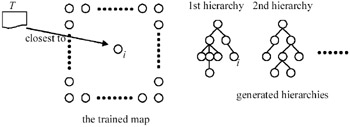Figure 3: The text categorization process.

The neighborhood Bi in calculating supporting cluster similarity of a neuron i may be arbitrarily selected. Two common selections are circular neighborhood and square neighborhood. In our experiments, the shapes of the neighborhood are not crucial. It is the sizes of the neighborhood, denoted by Nc1, that matter. Different sizes of neighborhoods may result in different selections of dominating neurons. Small neighborhoods may not capture the necessary support from similar neurons. On the other hand, without proper weighting, a large Nc1 will incorporate the support from distant neurons that may not be similar to the neuron under consideration. In addition, large neighborhoods have the disadvantage of costing much computation time.

Neighborhoods are also used to eliminate similar clusters in the super-cluster generation process. In each stage of the process, the neighborhood size, denoted by Nc2, has a direct influence on the number of dominating neurons. Large neighborhoods will eliminate many neurons and result in less dominating neurons. Conversely, a small neighborhood produces a large number of dominating neurons. We must decrease the neighborhood size when the process proceeds because the number of neurons under consideration is also decreased.

In Step 3 of the super-cluster generation process algorithm, we set three stop criterions. The first criterion stops finding super-clusters if there is no neuron left for selection. This is a basic criterion, but we need the second criterion which limits the number of dominating neurons to constrain the breadth of hierarchies. The lack of the second criterion may result in shallow hierarchies with too many categories in each level if the neighborhood size is considerably small. An extreme case happens when the neighborhood size is 0. In such a case, Step 2 of the algorithm will not eliminate any neuron. As a result, every neuron will be selected as dominating neurons, and we will obtain J single level hierarchies. Determining an adequate neighborhood size and a proper number of dominating neurons is crucial to obtaining an acceptable result. The third criterion constrains the depth of a hierarchy. If we allow a hierarchy having large depth, then we will obtain a set of "slimy" hierarchies. Note that setting large depths may cause no effect because the neighborhood size and the number of dominating neurons may already satisfy the stop criterion. An ad hoc heuristic rule used in our experiments is to determine the maximum depth d if it satisfies the following rule: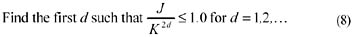where K is the dimension of the neighborhood and is defined as a ratio to the map's dimension. For example, if the map contains an 8 8 grid of neurons, K =4 means that the dimension of the neighborhood is one-fourth of the map's dimension, which is 2 in this case. The depth d that satisfies Eq. 8 is then 2.

Notice that there some "spare" neurons may exist that are not used in any hierarchy after the hierarchy generation process. These neurons represent document clusters that are not significant enough to be a dominating neuron of a super-cluster in any stage of the process. Although we can extend the depths in the hierarchy generation process to enclose all neurons into the hierarchies, sometimes we may decide not to do so because we want a higher document-cluster ratio, that is, a cluster that contains a significant amount of documents. For example, if all clusters contain very few documents, it is not wise to use all clusters in the hierarchies because we may have a set of hierarchies that each contains many nodes without much information. To avoid producing such over-sized hierarchies, we may adopt a different approach. When the hierarchies have been created and there still exist some spare neurons, we simply assign each spare neuron to its nearest neighbor. This in effect merges the document clusters associated with these spare neurons into the hierarchies. The merging process is necessary to achieve a reasonable document-cluster ratio.

### Automatic Category Theme Identification

In last section we showed how to obtain the category hierarchies from the DCM. In each category hierarchy, a leaf node represents an individual neuron as well as a category. In this subsection, we will show a method to assign each node in a hierarchy to a label and create a human-interpretable hierarchy. These labels reflect the themes of their associated nodes, that is, categories. Moreover, the label of a parent category in a hierarchy should represent the common theme of its child categories. Thus, the label of the root node of a hierarchy should represent the common theme of all categories under this hierarchy. Traditionally, a label usually contains only a word or a simple phrase to allow easy comprehension for humans. In this work, we try to identify category themes, i.e., category labels, by examining the WCM. As we mentioned before, any neuron i in the DCM represents a category and includes a set of similar documents. The same neuron in the WCM contains a set of words that often co-occur in such set of documents. Since neighboring neurons in the DCM contain similar documents, some significant words should occur often in these documents. The word that many neighboring neurons try to learn most should be the most important word and, as a result, the theme of a category. Thus, we may find the most significant word of a category by examining the synaptic weight vectors of its corresponding neuron and neighboring neurons. In the following, we will show how to find the category themes. Let Ck denote the set of neurons that belong to the kth super-cluster, or category. The category terms are selected from those words that are associated with these neurons in word cluster map. For all neurons jεCk, we select the n*th word as the category term if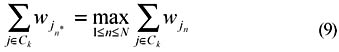where G: R+ + {0} R+ is a monotonically non-decreasing weighting function. G(j,k) will increase when the distance between neuron j and neuron k increases. The above equation selects the word that is most important to a super-cluster since the weight vector of a synaptic in a neuron reflects the willingness of that neuron to learn the corresponding input data, i.e., word. We apply Eq. 9 in each stage of the super-cluster generation process so when a new super-cluster is found, its theme is also determined. In STAGE-1, the selected themes label the root nodes of every category hierarchy. Likewise, the selected themes in STAGE-n are used as labels of the nth level nodes in each hierarchy. If a word has been selected in previous stages, it will not be a candidate of the themes in the following stages.

The terms selected by Eq. 9 form the top layer of the category structure. To find the descendants of these terms in the category hierarchy, we may apply the above process to each super-cluster. A set of sub-categories will be obtained. These sub-categories form the new super-clusters that are on the second layer of the hierarchy. The category structure can then be revealed by recursively applying the same category generation process to each newfound super-cluster. We decrease the size of neighborhoods in selecting dominating neurons when we try to find the sub-categories.

 Brought to you by Team-Fly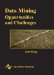Data Mining: Opportunities and Challenges
ISBN: 1591400511
EAN: 2147483647
Year: 2003
Pages: 194
Authors: John Wang